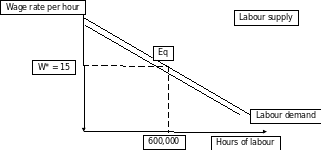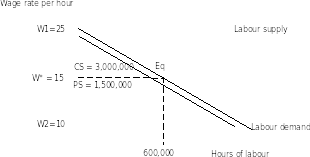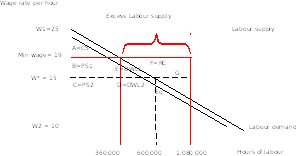# ECON111 Microeconomic Principles Paper Editing Services### ECON111 Microeconomic Principles Paper Editing Services

A minimum wage is the lowest amount of payment that the employer needs to pay to the employee (i.e. the wage earners) for a set period of time which cannot further be reduced by a set of contract law or agreement (Jardim et al. 2017). Binding minimum wage is set above the wage equilibrium level where the firms are forced to pay and are not willing to hire at this particular wage.

As per Australia’s national workplace relations tribunal (“Fair Work Commission”), the minimum wage rate for an adult is \$18.93 per hour and if calculated based on a week of 38 hours, then it would be \$719.20 per week (FWC 2018).

### To calculateequilibriumwage rate, labour demand and labour supply should be equal for hours of labour.

Here, Labour demand = Labour supply

è1,500,000 – 60,000W = 120,000W – 1,200,000

è 120,000W + 60,000W = 1,500,000 + 1,200,000

180,000 = 2,700,000è

W = 2,700,000/180,000 = 15è

Unskilled labour at the existing wage rate can be given as

=1,500,000 – 60,000W

= 1,500,000 – 60,000 * 15

= 1,500,000 – 900,000

= 600,000

Hence, equilibrium wage rate = \$15 with 600,000 as quantity of unskilled labourers

Diagrammatically, it can be presented as given below###D = 1,500,000 – 60,000W

The maximum a firm is prepared to pay to its workers is given by W1

?W1= 1,500,000/ 60,000 = 25

S = 120,000W – 1,200,000

The minimum a firm is supposed to pay as cost for labour is given by W2

?W2= 1,200,000/ 120,000 = 10

### Consumer Surplus or Firm’s Surplus = ½ * base * height

? Consumer Surplus = ½ * 600,000 * (25-15)

? Consumer Surplus = ½ * 600,000 * 10

?Consumer Surplus or Firm’s Surplus = ½ * 6,000,000 = 3,000,000

### Producer Surplus or worker’s Surplus = ½ * base * height

? Producer Surplus = ½ * 600,000 * (15-10)

? Producer Surplus = ½ * 600,000 * 5

? Producer Surplus or Worker’s Surplus = ½ * 3,000,000 = 1,500,000

### Total Surplus = Firm’s Surplus + Worker’s Surplus

? Total Surplus = 3,000,000 + 1,500,000

?Total Surplus = 4,500,000

### Part 5i)

When the FWC puts forward a minimum wage of \$19, the hours of labour exchanged in the market can be given as

Hours of Labour demand = 1,500,000 – 60,000W= 1,500,000 – 60,000 * 19 =1,500,000 – 1,140,000 =360,000

Hours of Labour Supply = 120,000W – 1,200,000 = 120,000 * 19 – 1,200,000 = 2,280,000 – 1,200,000 = 1,080,000

### Part 5ii)

At \$19, minimum wage, hours of supply labour exceeds demand.

The difference of Hours of Labour Supply – Hours of Labour demand = 1,080,000 – 360,000 = 720,000

###Calculation when minimum wage = \$19

### Consumer surplus = A =½ * base * height

? Consumer Surplus = ½ * 360,000 * (25 - 19)

? Consumer Surplus = ½ * 600,000 * 6

?Consumer Surplus or Firm’s Surplus = A = ½ * 3,600,000 = 1,080,000

### Producer Surplus or worker’s Surplus = B + C

? B = 360,000 * (19 - 15) = 360,000 * 4

? B = 1,440,000

C = {(C + D) – D}

?C = {( ½ * 600,000 * (15 - 10)) – ( ½ * (600,000- 360,000) * (15 - 13))}

?C = ( ½ * 600,000 * 5) – ( ½ * 240,000 * 2)

?C = (½ * 3,000,000) – (1* 240,000)

?C = 1,500,000 – 240,000

?C = 1,260,000

Producer Surplus or worker’s Surplus = B + C =1,440,000 + 1,260,000 = 2,700,000

### Total Surplus = Firm’s Surplus + Worker’s Surplus

? Total Surplus = 1,080,000 + 2,700,000

?Total Surplus = 3,780,000

### F = Resource Cost

Resource Cost= (E + F + G) – E – G

E + F + G= Length * breadth

?E + F + G = (1,080,000- 360,000) * (19 - 15) = (720,000 * 4) = 2,880,000

E =½ * base * height

?E = 0.5* (600,000 - 360,000) * (19 - 15) = ( ½ * 240,000 * 4) = 480,000

G =½ * base * height

?G = 0.5* (1,080,000 – 600,000) * (19 - 15) =( ½ * 480,000 * 4) = 960,000

Resource Cost = (E + F + G) – G – E = 2,880,000– 960,000 – 480,000 = 1,440,000

### D + E = Dead Weight Loss

?E = 0.5* (600,000 - 360,000) * (19 - 15) = ( ½ * 240,000 * 4) = 480,000

?D = 0.5* (600,000 - 360,000) * (15 - 13) = ( ½ * 240,000 * 2) = 240,000

Dead Weight Loss = E + D= 480,000 + 240,000 = 720,000

### Part 7i)

Firms better off position can be analysed from consumer’s surplus. After the imposition of minimum wage, the surplus has decreased to a greater amount by (3,000,000 – 1,080,000) = 1,920,000 stating the firm is in a worse off condition after the minimum wage.

### Part 7ii)

The worker’s have been enjoying the additional surplus after the imposition of minimum wage. The surplus after \$19 imposition has increased by (2,700,000 – 1,500,000) = 1,200,000 receiving a high wage per hour.

### Part 7iii)

The society has been facing a dead weight loss situation with resource lost. Earlier before minimum wage, the wage rate (\$15) was at equilibrium with no welfare loss. However, after imposition of minimum wage, there is dead weight loss of 720,000. Also, with the decline in firm’s surplus, the worker’s surplus has increased but shows a total decline in total surplus from 4,500,000 (\$15 wage rate) to 3,780,000 (\$19 minimum wage rate).

### Part 8i)

Consumer surplus = A =½ * base * height

? Consumer Surplus = ½ * 360,000 * (25 - 19)

? Consumer Surplus = ½ * 600,000 * 6

?Consumer Surplus or Firm’s Surplus = A = 1,080,000 (it remains the same as it is)

### Earlier Producer Surplus or worker’s Surplus = B + C = 1,440,000 + 1,260,000 = 2,700,000

Resource Cost = (E + F + G) – F – E= 2,880,000– 960,000 – 480,000 = 1,440,000

DWL = E + D = 480,000 + 240,000 = 720,000

Total Worker’s Surplus = B + C + (E + F + G) – F – E + E + D = B + C + G + D = 2,700,00 + 1,440,000 + 720,000 = 4,860,000

### Total Surplus = Firm’s Surplus + Worker’s Surplus

? Total Surplus = 1,080,000 + 4,860,000

?Total Surplus = 5,940,000

### Part 8iv)

No DWL as it is considered under the worker’s surplus

The calculation done in part 7 change from part 8. This is because as the resource cost in job is considered to be under worker’s surplus. It creates an additional surplus for the workers with no change in firm’s surplus. Moreover, the total surplus increases by increasing social welfare in the economy.

Ethically, setting up of higher minimum wage was the means to reduce unemployment and to fire the unproductive workers. As a result, it started creating allocative inefficiencies by not distributing the equality of marginal benefit and marginal cost such that the employers paying the wage should be willingly accepted by the workers for a fixed period (Cothren and Radhakrishnan 2018). However, this willingness was lost for the skilled labourers creating a disparity in labour demand and supply (Altman 2016). The marginal cost of labour supply was less than the marginal benefit received by workers. In addition, it not only hampered the welfare of the economy but also created inequality in wages.

The workers receive extra benefit when the minimum wage rate is imposed by the government. As there is increase in wage, the workers supply with more hours of labour and the firm is directed to hire for labour with a higher level of cost (Atkinson and Storey 2016). However, creating excess labour supply in the market further leading to unemployment. Although, the worker’s standard of living improves at the minimum wage rate but due to hiring restriction by the firms; the workers who get unemployed or remain unemployed suffer dearly by creating additional reserve of job resource cost (Ehrenberg and Smith 2016). It is not ethically justified, that the existing unskilled labourers have higher standard of living by neglecting the social welfare in the economy only by a portion of workers.

Minimum wage that is binding in nature leads to disequilibrium as the market is diminished by demand followed by excess supply of labour creating allocating inefficiencies. Moreover, it is not considered to be an appropriate tool for redistribution (Gerritsen and Jacobs 2016). The minimum wage set by the government is to maintain standard of living but it oversimplifies not only the unskilled labour market but marks to generate equivalent productivity (Jardim 2017). However, for unskilled workers the binding wage rate can be considered as a boon but for skilled labourers is the restriction imposed on their capabilities.

Without the minimum wage rate, when the economy is operating with the forces of demand and supply, the equilibrium doesn’t distinguishes between skilled and unskilled labourers. Also, it creates unemployment in the economy as the firm is not ready to hire more unskilled labourers at this binding rate. As a result, the skilled labourers might lose their job further creating a decline in surplus for the economy.

#### References

1. "National Minimum Wage Orders". 2018. FWC. https://www.fwc.gov.au/awards-and-agreements/minimum-wages-conditions/national-minimum-wage-orders.
2. Altman, Morris. "The living wage, economic efficiency, and socio-economic wellbeing in a competitive market economy." In Forum for Social Economics, vol. 41, no. 2-3, pp. 166-186. Routledge, 2012.
3. Atkinson, John, and David J. Storey. Employment, the small firm and the labour global market. Routledge, 2016.
4. Cothren, Richard, and Ravi Radhakrishnan. "Productivity growth and welfare in a model of allocative inefficiency." Journal of Economics 123, no. 3 (2018): 277-298.
5. Ehrenberg, Ronald G., and Robert S. Smith. Modern labor economics: Theory and public policy. Routledge, 2016.
6. Gerritsen, Aart, and Bas Jacobs. "Is a minimum wage an appropriate instrument for redistribution?." (2016).
7. Jardim, Ekaterina, Mark C. Long, Robert Plotnick, Emma Van Inwegen, Jacob Vigdor, and Hilary Wething. Minimum wage increases, wages, and low-wage employment: Evidence from Seattle. No. w23532. National Bureau of Economic Research, 2017.